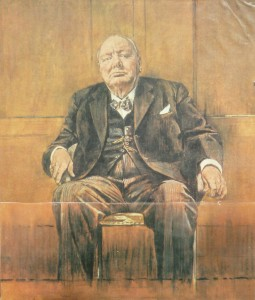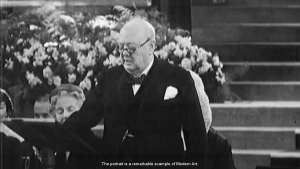## 另外一种定义欧拉数$$e$$的方法

$$e=\lim_{n \to \infty} (1+\frac{1}{n})^n$$

$$f’=f$$                         ……（1）

$$f(x+a)=c*f(x)$$                     ……（2）

$$f(x+a)=f(a)*f(x)$$

$$f(1)=exp(1)=1+1+\frac{1}{2!}+\frac{1}{3!}+…+\frac{1}{n!}+…$$

$$\begin{gather*} \frac{d f(x)}{d x} = f(x)\\f(0)=1 \end{gather*}$$

$$\frac{d^2I}{dt^2}=-cI$$，其中$$c$$为大于零的常数。

$$k^2*e^{k*t}=-c*e^{k*t}$$，因此，

$$k^2=-c\\k=\pm\sqrt{c}*i$$

The reason this number e is considered the “natural” base of exponential functions is that this function is its own derivative.

This natural exponential function is identical with its derivative. This is really the source of all the properties of the exponential function, and the basic reason for its importance in applications…

## Another documentary from Simon Schama

I’m a big fan of <A history of Britain> and <Power of Art>, so inevitably became of fan of Simon Schama.

Face of Britain is the new documentary from Simon Schama. I just watched the 1st part, Face of Power. It’s just as brilliant as the other Simon Schama documentaries. In particular, I like Simon Schama’s way of story telling. For example, here’s the opening story of the first part.On Churchill’s 80th birthday on 1954, the painting commissioned a portrait for him as a present to proclaim his contribution and leadership during WWII.

By the time the parliament is about to unveil this painting in an formal assemble, Churchill had already seen it and hated it. Here’s how he remarked:The portrait is a remarkable example of modern art.

Churchill hated this painting so much that in the end this painting was burned.

Wikipedia has a page for this story, but it was found out rather recently that the painting was burnt soon after the event.

Simon Schama uses this story to show the “will contest” between the painter and the person being portrayed. I was fascinated instantly.